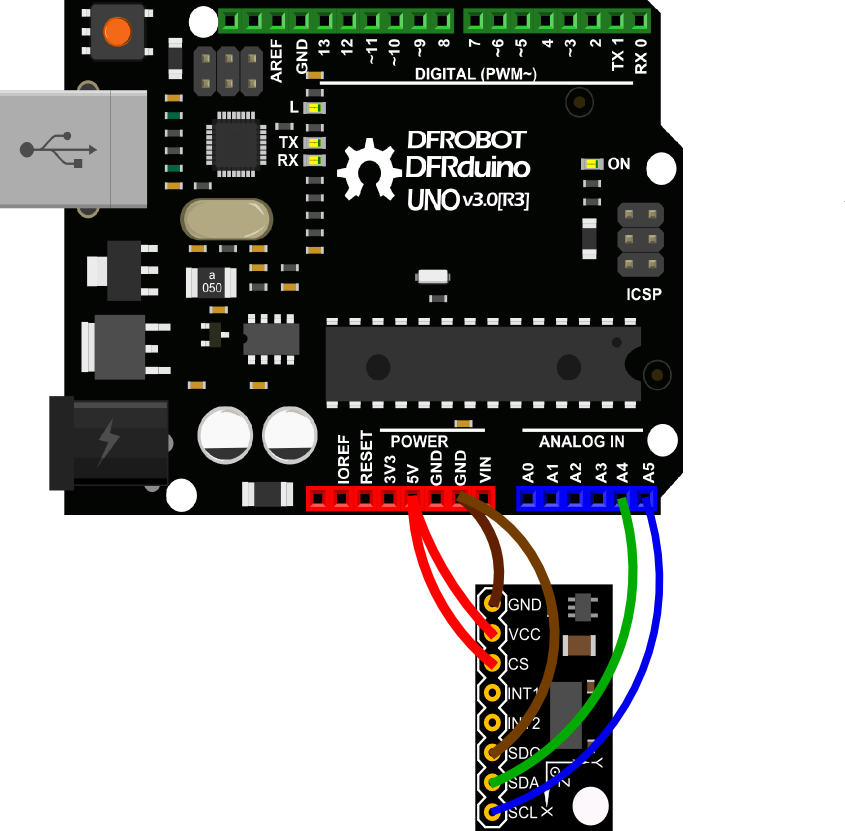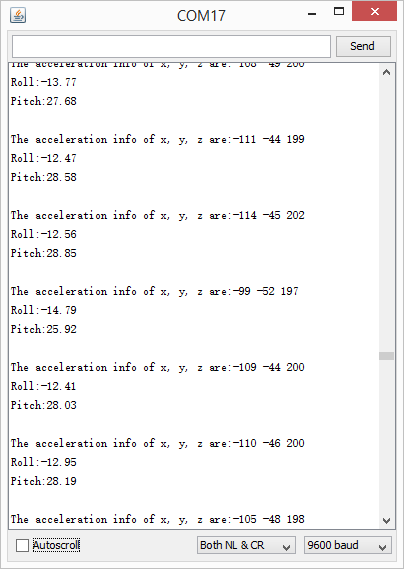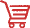## Introduction

Breakout board for the Analog Device ADXL345. The ADXL345 is a small, thin, low power, 3-axis accelerometer with high resolution (13-bit) measurement at up to ±16 g. Digital output data is formatted as 16-bit twos complement and is accessible through either a SPI (3- or 4-wire) or I2C digital interface. The ADXL345 is well suited to measures the static acceleration of gravity in tilt-sensing applications, as well as dynamic acceleration resulting from motion or shock. Its high resolution (4 mg/LSB) enables measurement of inclination changes less than 1.0°.

Several special sensing functions are provided. Activity and inactivity sensing detect the presence or lack of motion and if the acceleration on any axis exceeds a user-set level. Tap sensing detects single and double taps. Free-fall sensing detects if the device is falling. These functions can be mapped to one of two interrupt output pins. An integrated, patent pending 32-level first in, first out (FIFO) buffer can be used to store data to minimize host processor intervention. Low power modes enable intelligent motion-based power management with threshold sensing and active acceleration measurement at extremely low power dissipation.

## Specification

• Working voltage: 3.3~6V
• Current consumption @2.5v: 40uA / working mode, 0.1uA / standby mode
• Communication interface: I2C / SPI (3 or4 lines)
• Size: 20x15mm

## Application

• Tap/Double Tap Detection
• Free-Fall Detection
• Selecting Portrait and Landscape Modes
• Tilt sensing

## Connection Diagram

This diagram is an IIC connection method suitable with Arduino UNO. It would be differen if you use other Arduino Controllers which the SCL & SDA pin might be different. And if you want to use SPI interface, please refer to ADXL345 datasheet for more info.## Sample Code

Upload the sample sketch bellow to UNO or your board to check the 3-axis acceleration data and the module's tilt information.

For Arduino

``````#include <Wire.h>

#define TO_READ (6)        //num of bytes we are going to read each time (two bytes for each axis)

byte buff[TO_READ] ;        //6 bytes buffer for saving data read from the device
char str;              //string buffer to transform data before sending it to the serial port
int x, y, z;                        //three axis acceleration data
double roll = 0.00, pitch = 0.00;       //Roll & Pitch are the angles which rotate by the axis X and y
// https://www.dfrobot.com/wiki/index.php?title=How_to_Use_a_Three-Axis_Accelerometer_for_Tilt_Sensing#Introduction

void setup() {
Wire.begin();         // join i2c bus (address optional for master)
Serial.begin(9600);  // start serial for output

writeTo(DEVICE, 0x2D, 0);
writeTo(DEVICE, 0x2D, 16);
writeTo(DEVICE, 0x2D, 8);
}

void loop() {

//each axis reading comes in 10 bit resolution, ie 2 bytes.  Least Significat Byte first!!
//thus we are converting both bytes in to one int
x = (((int)buff) << 8) | buff;
y = (((int)buff)<< 8) | buff;
z = (((int)buff) << 8) | buff;

//we send the x y z values as a string to the serial port
Serial.print("The acceleration info of x, y, z are:");
sprintf(str, "%d %d %d", x, y, z);
Serial.print(str);
Serial.write(10);
//Roll & Pitch calculate
RP_calculate();
Serial.print("Roll:"); Serial.println( roll );
Serial.print("Pitch:"); Serial.println( pitch );
Serial.println("");
//It appears that delay is needed in order not to clog the port
delay(50);
}

//---------------- Functions
//Writes val to address register on device
void writeTo(int device, byte address, byte val) {
Wire.beginTransmission(device); //start transmission to device
Wire.write(val);        // send value to write
Wire.endTransmission(); //end transmission
}

//reads num bytes starting from address register on device in to buff array
Wire.beginTransmission(device); //start transmission to device
Wire.endTransmission(); //end transmission

Wire.beginTransmission(device); //start transmission to device
Wire.requestFrom(device, num);    // request 6 bytes from device

int i = 0;
while(Wire.available())    //device may send less than requested (abnormal)
{
i++;
}
Wire.endTransmission(); //end transmission
}

//calculate the Roll&Pitch
void RP_calculate(){
double x_Buff = float(x);
double y_Buff = float(y);
double z_Buff = float(z);
roll = atan2(y_Buff , z_Buff) * 57.3;
pitch = atan2((- x_Buff) , sqrt(y_Buff * y_Buff + z_Buff * z_Buff)) * 57.3;
}``````

For Micropython

``````from machine import Pin,I2C
import time

i2c = I2C(scl=Pin(22),sda=Pin(21), freq=10000)

while True:
print('The acceleration info of x, y, z are:%d,%d,%d'%(x,y,z))
Open the Serial monitor to see the 3-axis acceleration data and Roll-Pitch angle. See changs as you sway the Accelerometer.Get Triple Axis Accelerometer Breakout - ADXL345 (SKU:SEN0032) from DFRobot Store or DFRobot Distributor.